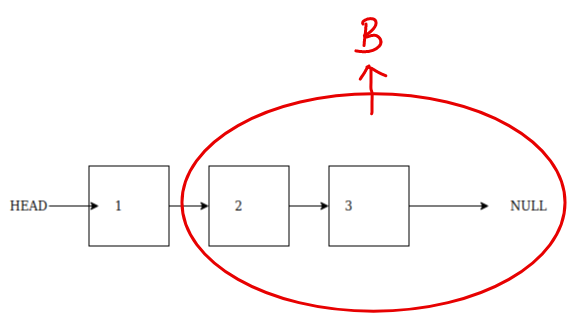# How to recursively print a linked list ?

## How to recursively print a linked list ?

Linked List is a linear data structure in which each node points to the next node in the linked list.
Linked List is also a recursive data structure, for example we can say that each node of a linked list points to another linked list as shown in the diagrams below:Linked list thought of normally

We can also think of the above linked list as this:Linked List thought recursively

When we think of the linked list as recursive data structure, as shown in the diagram above. We can say that Node 1 points to another linked list (B) of size 2, similarly node 2 points to a linked list of size 1, node 3 points to a linked list of size 0
This observation will help us print the linked list recursively

Therefore, to print this linked list recursively , we will write a function which prints the current node first and then prints the remaining Linked List (B) recursively.

``````// curr is the current node that we want to print
void printLinkedListRecursively(Node* curr) {
if (curr != NULL) {
cout << curr->data << " ";
printLinkedList(curr->next);
}
}

//We will call the above function as
printLinkedList(head);
``````
Scroll to Top

### Full Stack Integrated Bootcamp Free Trial

• Please enter a number from 7000000000 to 9999999999.